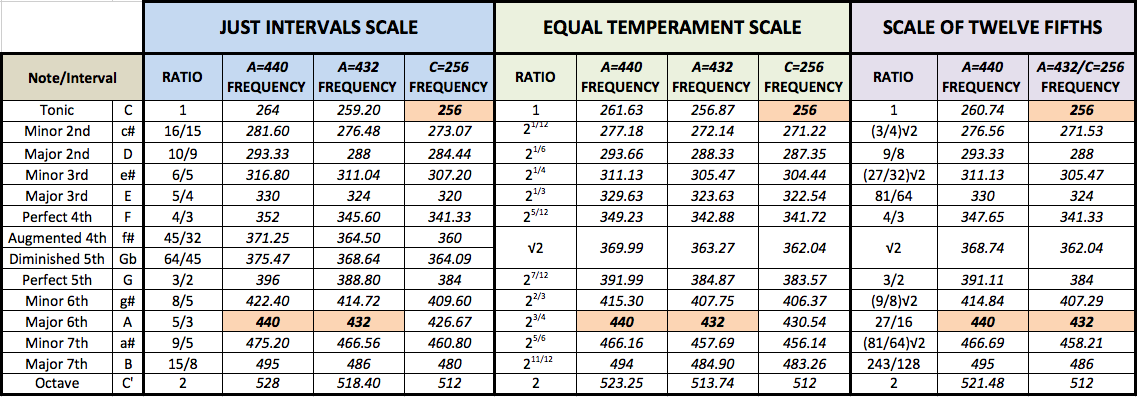# Pitch change effect and scale of fifths

Hello,
i would like to ask about some matter, more precisely about how tone changing of notes works in audacity plugin “change pitch”. I know audacity operates on 12 note equal temperament scale (where interval between each of 12 notes is constant (equal to pow(2, 1/12)) so for example: E4 = 329.63Hz so F4 would be equal to E4 * pow(2, 1/12), so F4 = 329.63 Hz * 1.0595; F4 = 349.23Hz etc. of course everything provided yet in equal temperament scale, same as audacity plugin works (and same as standard).

To the point:
I extracted from source files code of audacity 2.0.5 (ChangePitch.cpp and headers) and i need to know where (how) are calculated specific tones. I know things or two about c++ but i couldn’t yet find function which is responsible for calculating those frequencies. Btw i’m on linux but here it doesn’t matter i suppose. So the question is where is function which calculates specific note frequency in pitch change effect?

What i want to do:
“convert” equal temperament scale to scale of twelve fifths. I will handle calculations, need only to get answer for question provided. Anyway - help will be greatly appreciated.

Look at line 460 in ChangePitch.cpp for the comment:

``````// calculations
``````

The calculation functions are below that.
In particular, look at

``````void ChangePitchDialog::Calc_SemitonesChange_fromPercentChange()
``````

Now i’m looking for information where

``````m_dPercentChange
``````

have it’s origins.

Got this clue but not sure if its legit:

``````m_dPercentChange = dlog.m_dPercentChange;
``````

The actual stretching is performed by SoundTouch (http://www.surina.net/soundtouch/)
Audacity passes m_dSemitonesChange to SoundTouch (line 171).

The “Pitch”, “Semitones”, “Frequency (Hz)” and “Percent Change” in the Change Pitch effect are interactive so that when one is changed it updates the others, which is handled in the “calculations” code.

A problem that you will have in converting to Pythagorean tuning (which I assume is what you mean by “scale of twelve fifths”) is that the percentage change between semitones varies depending on which tone of the scale you are changing. Really you will need an additional control to set the root (key) note from which you can calculate the required percentage change for each semitone (possibly as a lookup table).I did all calculations needed. I just need to know:
does i need to make any change in audacity sorce code formulas or correcting soundtouch will do the job?

If you work out from your “ratio” column what the “percent change” is for each interval, then you don’t need to make any changes at all - just use the “Percentage” input in the Change Pitch".

But this does only work for monophonic sounds with a stationary pitch, doesn’t it?
You should instead use something like auto-tune, where you can adjust the cent slider for each note.

indeed. The thing is - i need to edit formula of frequency calculation for each note, because its not constant like in equal temperament scale (difference is 1.059… , more precisely pow(2, 1/12). In monophonic sounds (frequencies) just pitch changing would be ok, but not in the case of music

So you realise that this is not going to work for polyphonic music? If two or more notes play at the same time you will not be able to adjust the pitch of one note without also adjusting the pitch of all notes that are playing at that time. For example, referring to your tuning chart, if there is an A4 and a C5 playing at the same time, in A440 equal temperament the frequencies are 440 Hz and 523.25 Hz. If you stretch the pitch so that the C 5 becomes 521.48 Hz, then the A 4 will no longer be 440 Hz.

Soundtouch plugin would need modification, but audacity plugin most likely needs to be written from zero. I’m aware of that. And i wonder what will happen with frequency detection